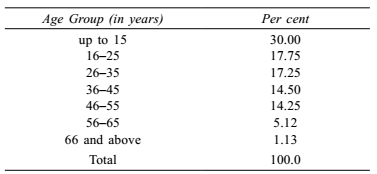## Table chart

#### Data Interpretation

Direction: A Table showing the percentages of the total population of a State by age groups for the year 1991 is given below. Answer the questions given below it.1. Out of every 4200 persons, the number of persons below 26 years is:

1. Required number = percent of persons below 26 years x total number of persons

##### Correct Option: A

As per above given table , we have
Given :- total number of persons = 4200
percent of persons below 26 years = ( 30 + 17.75 ) = 47.75%
Required number = percent of persons below 26 years x total number of persons

 = 4200 x 47.75 = 2005.50 = 2006 approx 100

1. If there are 10 million people in the age group 56 years and above, what is the difference between the number of people in the age groups 16–25 and 46–55?

1. Given :- difference between the Percent of people in the age groups 16–25 and 46–55 = 3.50%
Required. difference in the population

 = Total number of people in the age group 56 years and above x 3.50 percentage of people in the age group 56−65

##### Correct Option: A

Given :- Difference between the Percent of people in the age groups 16–25 and 46–55 = 3.50
percentage of people in the age group 56−65 = 5.12%
Total number of people in the age group 56 years and above = 10 million
Required difference in the population

 = Total number of people in the age group 56 years and above x 3.50 percentage of people in the age group 56−65

 = 10 x 3.50 ≈ 6.8 million 5.12

1. There are 200 million people below 36 years. How many million (approx) people are in the age group 56–65?

1. ∵ Number of people in the age group 56 − 65

 = Total number of people age below 36 years x percentage of people in the age group 56−65 65

##### Correct Option: B

Given :- percentage of people in the age group 56−65 = 5.12
Total number of people age below 36 years = 200 million
∵ Number of people in the age group 56−65

 = Total number of people age below 36 years x percentage of people in the age group 56-65 65

 = 200 x 5.12 ≈ 15.75 65

1. If the difference between the number of people in the age groups 46–55 and 26–35 is 11.75 million, then total population of State is approximately

1.  Total population = difference between the number of people in the age groups 46–55 and 26–35 x 100 difference between the percentage of people in the age groups 46–55 and 26–35

##### Correct Option: B

Given :- difference between the number of people in the age groups 46–55 and 26–35 = 11.75 million
difference between the percentage of people in the age groups 46–55 and 26–35 = 17.25 - 14.25 = 3 million

 Total population = difference between the number of people in the age groups 46–55 and 26–35 x 100 difference between the percentage of people in the age groups 46–55 and 26–35

 = 11.75 x 100 ≈ 391.67 million 3

1. Which age group accounts for the maximum population in the State?

1. Maximum population shows the age group up to 15 years, but among the options it is not there.

##### Correct Option: A

From above given table ,
Maximum population shows the age group up to 15 years, but among the options it is not there.
Hence, among the given options age group 16 − 25 is responsible for maximum population.